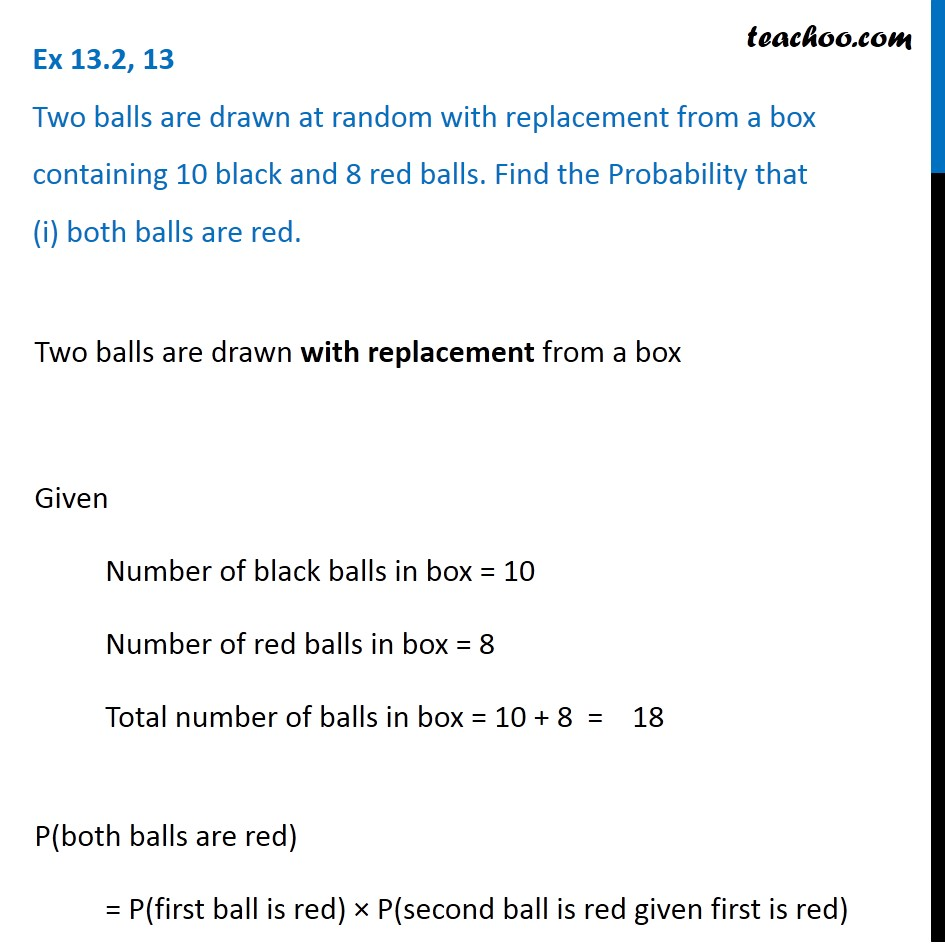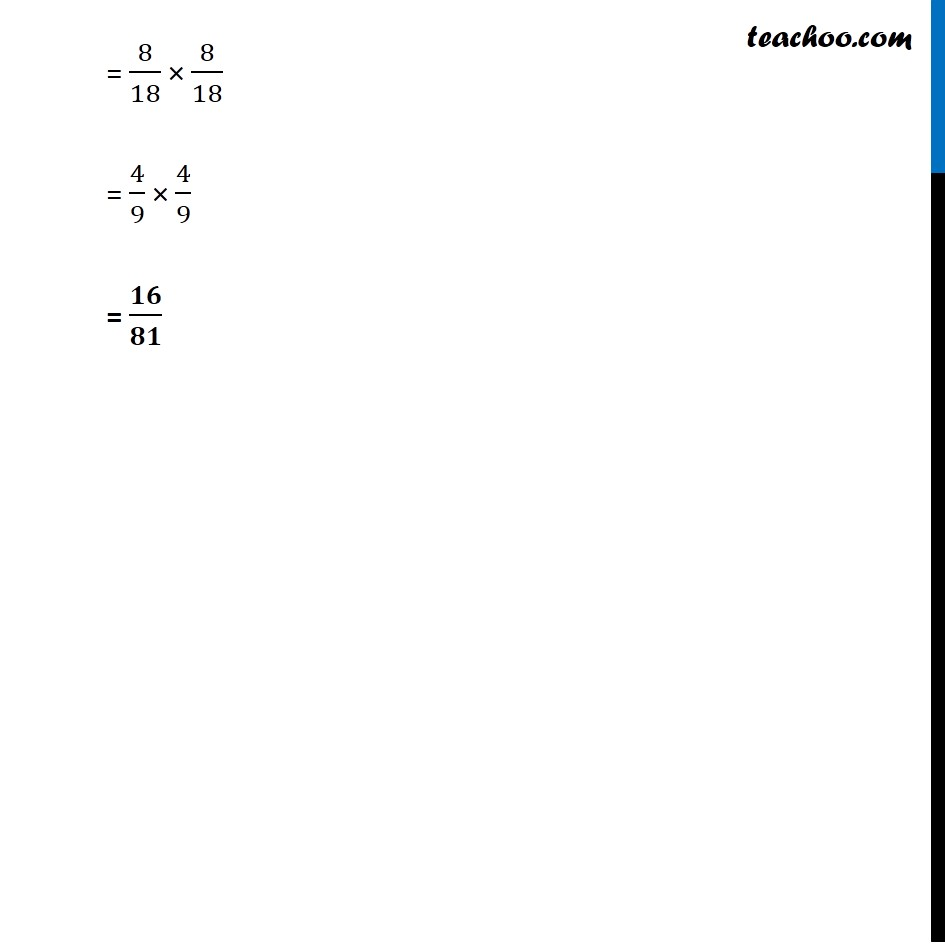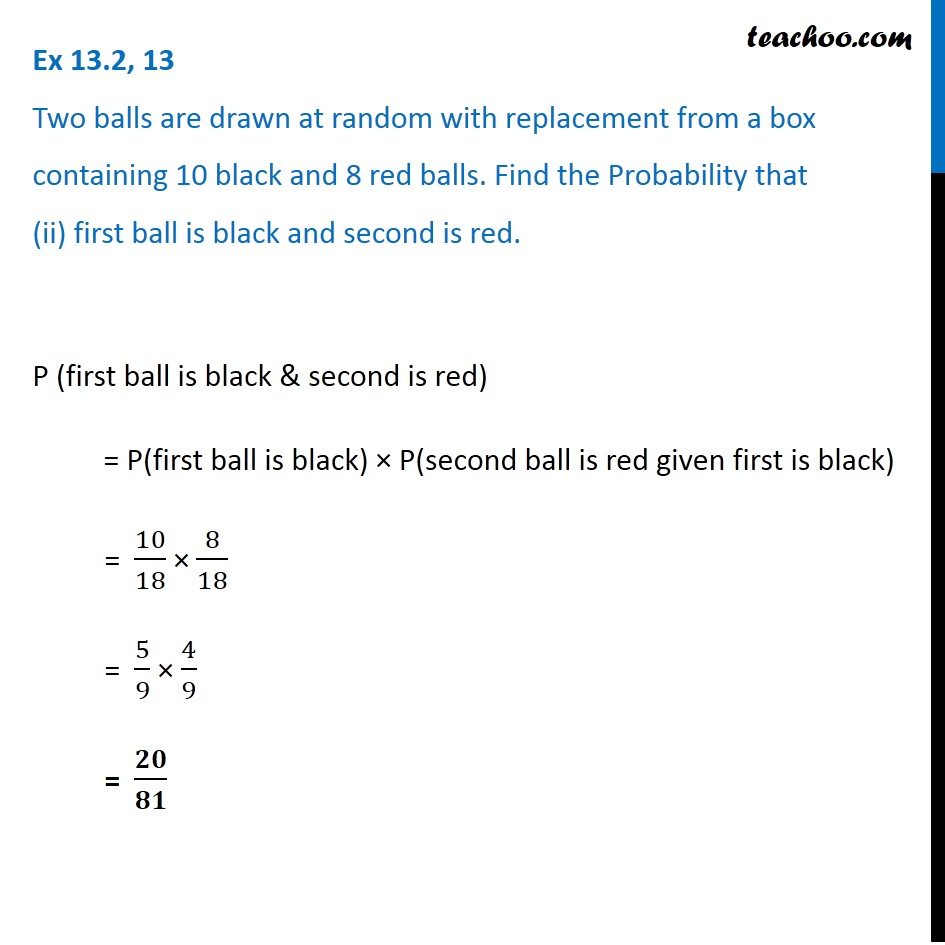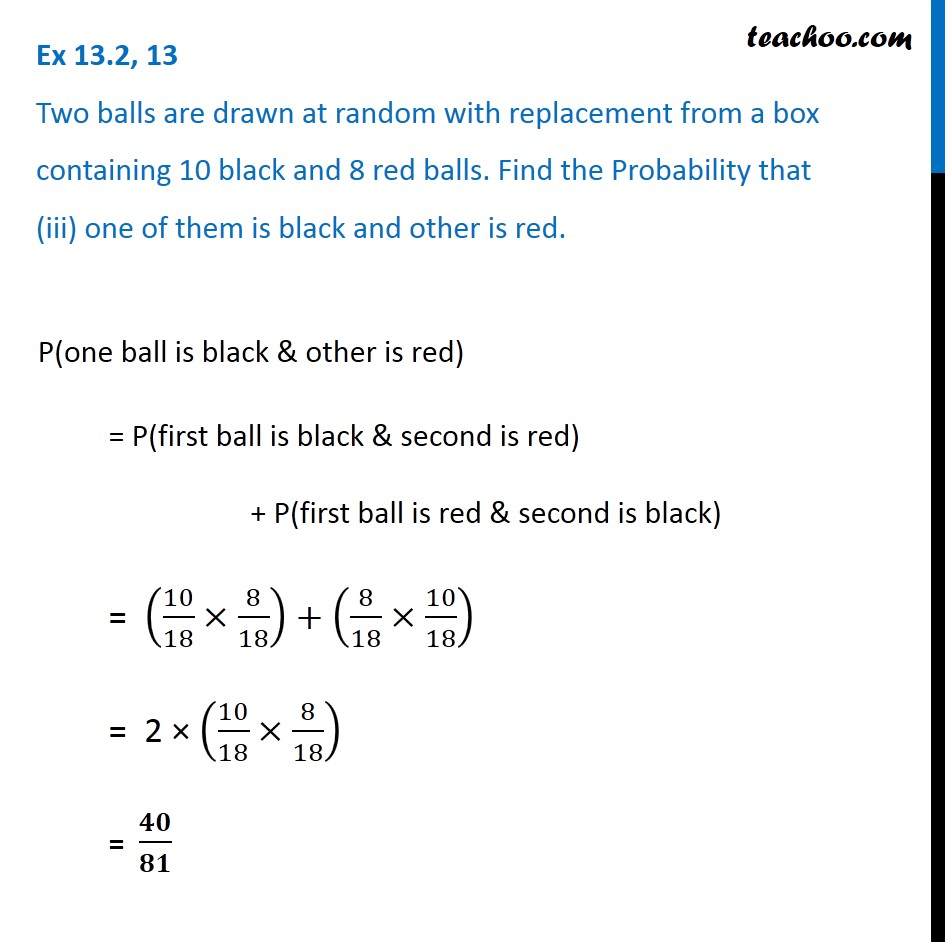Ex 13.2

Chapter 13 Class 12 Probability
Serial order wiseLearn in your speed, with individual attention - Teachoo Maths 1-on-1 Class

### Transcript

Ex 13.2, 13 Two balls are drawn at random with replacement from a box containing 10 black and 8 red balls. Find the Probability that (i) both balls are red.Two balls are drawn with replacement from a box P(both balls are red) = P(first ball is red) × P(second ball is red given first is red) = 8/18 × 8/18 = 4/9 × 4/9 = 𝟏𝟔/𝟖𝟏 Ex 13.2, 13 Two balls are drawn at random with replacement from a box containing 10 black and 8 red balls. Find the Probability that (ii) first ball is black and second is red.P (first ball is black & second is red) = P(first ball is black) × P(second ball is red given first is black) = 10/18 × 8/18 = 5/9 × 4/9 = 𝟐𝟎/𝟖𝟏 Ex 13.2, 13 Two balls are drawn at random with replacement from a box containing 10 black and 8 red balls. Find the Probability that (iii) one of them is black and other is red.P(one ball is black & other is red) = P(first ball is black & second is red) + P(first ball is red & second is black) = (10/18×8/18)+(8/18×10/18) = 2 × (10/18×8/18) = 𝟒𝟎/𝟖𝟏